# GSEB Solutions Class 12 Maths Chapter 13 Probability Miscellaneous Exercise

Gujarat Board GSEB Textbook Solutions Class 12 Maths Chapter 13 Probability Miscellaneous Exercise Textbook Questions and Answers.

## Gujarat Board Textbook Solutions Class 12 Maths Chapter 13 Probability Miscellaneous Exercise

Question 1.
A and B are two events such that P(A) ≠ ∅. Find P(B/A), if
(i) A is a subset of B
(ii) A ∩ B = ∅
Solution:
(i) A is a subset of B.
⇒ A ∩ B = A.Question 2.
A couple has two children.
(i) Find the probability that both children are males, if it is known that at least one of the children is male.
(ii) Find the probability that both children are female, if it is known that the elder child is a female.
Solution:
(i) Let the events A and B are denoted as
A = Both children are male, i.e., {MM}

B = At least one child is male, i.e; [MF, FM, MM]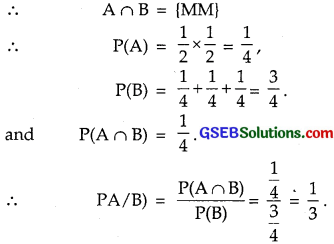(ii) A = Both the children are females = {FF}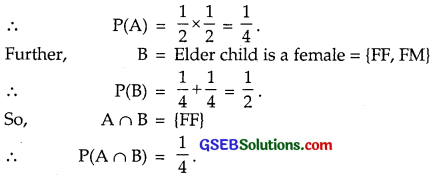P(Both the children are females, if elder child is female)Question 3.
Suppose that 5% of men and 0.25% of women have grey hair. A grey haired person is selected at random. What is the probability of this person being a male?
Assume that there are equal number of males and females?
Solution:
Probability, of selecting a male = P(E1) = $$\frac{1}{2}$$.
Probability of selecting a female = P(E2) = $$\frac{1}{2}$$.
5% men are grey haired.
⇒ P(A/E1) = 5% = 0.05.
0. 25% women have grey hair.
i.e. P(E1/A) = 0.25% = 0.0025.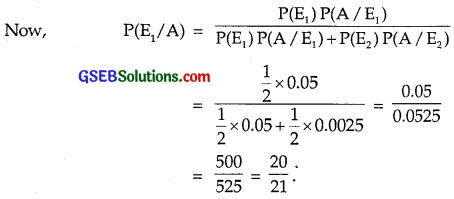Question 4.
Suppose that 90% of people are right handed. What is the probability that at most 6 of a random sample of 10 people are right handed?
Solution:
Right handed persons are 90%.
So, p = $$\frac{9}{10}$$.
Left handed persons are 10%.
⇒ q = $$\frac{1}{10}$$.
Here, n = 10
P(At most 6 persons are right handed)
= P(0) + P(1) + ……………. + P(6)
= [P(0) + P(1) + P(2) + …. + P(10)] – [P(7) + P(8) + P(9) + P(10)]
= 1 – [P(7) + P(8) + P(9) + P(10)]Question 5.
An urn contains 25 balls of which 10 bear a mark ‘X’ and the remaining 15 bear a mark ‘Y’. A ball is drawn at random from the urn, its mark noted down and it is replaced.
If 6 balls are drawn in this way, find the probability that
(i) all will bear mark ‘X’.
(ii) not more than two will bear ‘Y’ mark.
(iii) at least one ball will bear ‘Y’ mark.
(iv) the number of balls with ‘X! mark and ‘Y’ mark will be equal.
Solution:
Total number of balls = 25.
Number of balls marked ‘X’ = 10.
Let X denote the event of getting a ball marked X.
Y = event of getting a ball marked Y.
∴ P(X) = $$\frac{10}{25}$$ = $$\frac{2}{5}$$ = p
and P(Y) = 1 – $$\frac{2}{5}$$ = $$\frac{3}{5}$$ = q.
Now, 6 balls are drawn.
(i) P(all the balls are marked ‘X’) = ($$\frac{2}{5}$$)6
(ii) Event that not more than 2 will bear ‘Y’ mark)
⇒ (6X, 0Y), (5X, 1Y), (4X, 2Y)
∴ P(not more than 2 will bear the mark ‘Y’)
= P(6) + P(5) + P(4)(iii) Event that at least 1 ball will bear ‘Y’ mark
= {(5X, 1Y, (4X, 2Y), (3X, 3Y), (2X, 4Y), (X, 5Y), (0X, 6Y))
∴ P(at least 1 ball will bear mark Y)
= 1 – P(no ball will bear mark Y)
= 1 – P(all balls bear mark X)
= 1 – ($$\frac{2}{5}$$)6.

(iv) P(3 balls each are marked X and Y)Question 6.
In a hurdle race, a player has to cross 10 hurdles and the probability that he will clear each hurdle is $$\frac{5}{6}$$.
What is the probability that he will clear each hurdle is $$\frac{5}{6}$$. What is the probability that he will knock down fewer than 2 hurdles?
Solution:
There are 10 hurdles in all, i.e; n = 10.
He clears a hurdle, the probability is $$\frac{5}{6}$$.
∴ Probability that he does not clear the hurdle = 1 – $$\frac{5}{6}$$ = $$\frac{1}{6}$$.
P(knowing down fewer than 2)
= P(he will cross all hurdles) + P(he will not cross 1 hurdle)Question 7.
A dice iš thrown again and again until three sixes are obtained. Find the probability of obtaining the third six in the sixth throw of the dice.
Solution:
A dice is thrown again and again.
Probability of getting a ‘six’ in a throw = $$\frac{1}{6}$$.
∴ Probability of not getting a ‘six’ in a throw = 1 – $$\frac{1}{6}$$ = $$\frac{5}{6}$$.
There are two ‘sixes’ in first five throws and one ‘six’ at the sixth throw.Question 8.
If a leap year is selected at random, what is the chance that it will contain 53 tuesdays?
Solution:
A leap year has 366 days which contain 52 full weeks and 2 extra days. The extra days may occur as (Mon, Tue), (Tue, Wed), (Wed, Thu), (Thu, Fri), (Fri, Sat), (Sat, Sun), (Sun, Mon).
Now, the favourable cases are (Mon, Tue), (Tue, Wed)
∴ Probability that a leap year will have 53 Tuesdays = $$\frac{2}{7}$$.

Question 9.
An experiment succeeds twice as often as it fails. Find the probability that in the next six trials, there will be at least 4 successes.
Solution:
Let p denotes the probability of success and q denote the probability of a failure.
An experiment succeeds twice as often as it fails.
⇒ p = 2q = 2 (1 – p) = 2 – 2p
∴ 3p = 2 or p = $$\frac{2}{3}$$ ∴ q = $$\frac{1}{3}$$.
Probability that in six trials, there are at least 4 successes
= P(4) + P(5) + P(6)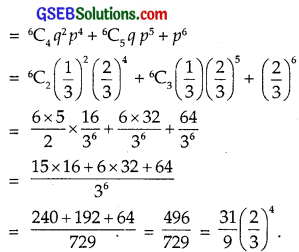Question 10.
How many times must a man toss a fair coin so that the probability of having at least one head is more than 90%?
Solution:
Let the coin is tossed in n times.
Probability of getting a head = $$\frac{1}{2}$$.
∴ Probability of getting no head in tossing a coin n times = ($$\frac{1}{2}$$)n
∴ Probability of getting at least one head = 1 – ($$\frac{1}{2}$$)n ………………….. (1)
We have probability of getting at least one head 90% = 0.9 …………….. (2)
From (1) and (2)Question 11.
In a game, a man wins a rupee for a six and looses a rupee for any other number, when a fair dice is thrown.
The man decided to throw a dice thrice but to quit as and when he gets a six. Find the expected value of the amount he wins/looses.
Solution:
When a dice is thrown, probability of getting a six
= $$\frac{1}{6}$$ = p(say)
∴ q = 1 – $$\frac{1}{6}$$ = $$\frac{5}{6}$$
(i) If he gets a six in first throw, then probability of getting a six = $$\frac{1}{6}$$.
(ii) If he does not get a six in first throw but he gets a six in second throw, its probability = $$\frac{5}{6}$$ × $$\frac{1}{6}$$ = $$\frac{5}{36}$$.
Probability that he does not get a six in first two throws and he get a six in third throw
= $$\frac{5}{6}$$ × $$\frac{5}{6}$$ × $$\frac{1}{6}$$ = $$\frac{25}{216}$$
Probability that he does not get a six in any of the three throws
= ($$\frac{5}{6}$$)3 = $$\frac{125}{216}$$.
If in first throw, he gets a six, he will received ₹1.
If he gets a six in second throw, he will receive ₹(1 – 1)
= ₹0
If he gets a six in third throw, he will receive
= ₹(- 1 – 1 + 1) = ₹(- 1) = he will lose ₹1
Expected valueExpected value is, he will win ₹$$\frac{11}{216}$$.Question 12.
Suppose we have four boxes A, B, C and D containing coloured marbles as given below:One of the boxes has been selected at random and a single marble is drawn from it. If the marble is red, what is the probability that it was drawn from box A? box B? box C?
Solution:
Let F be the event of selecting a box and A be the selection of a red ball.
P(F1) = P(F2) = P(F3) = P(F4) = $$\frac{1}{4}$$.
Each box has 10 marbles. Boxes A, B, C and D contain 1, 6, 8 and 0 red marbles respectively.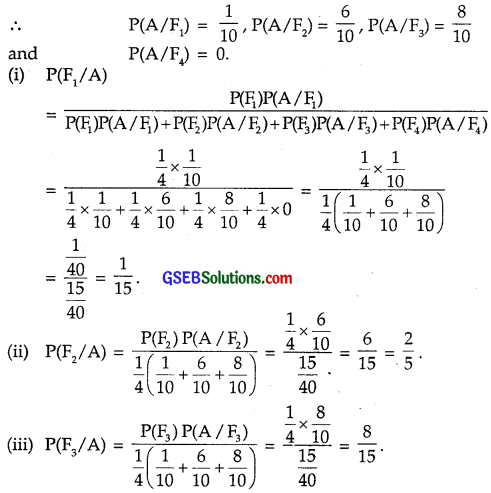Hence, the probability when a red ball is drawn :
Selecting the Box A = $$\frac{1}{15}$$; Selecting the Box B = $$\frac{2}{5}$$; Selecting the Box C = $$\frac{8}{15}$$.

Question 13.
Assume that the chance, of a patient having a heart attack 40%. It is also assumed that a meditation and yoga course reduces the risk of heart attack by 30% and prescription of a certain drug reduces its chance by 25%.
At a time, a patient can choose any one of the two options with equal probabilities. It is given that after going through one of the two options, the patient selected at random suffers a heart attack.
Find the probability that the patient followed a course of meditation and yoga.
Solution:
A patient has options to have the treatment of yoga and meditation and that of prescription of drugs.
Let these events be denoted by E1 and E2, i.e.,
E1 : Treatment of Yoga and Meditation
and E2 : Treatment of prescription of certain drugs.
Now, P(E1) = $$\frac{1}{2}$$, P(E2) = $$\frac{1}{2}$$
Let A denotes that a person has heart attack.
So, P(A) = 40% = 0.40.
Yoga and Meditation reduces the heart risk by 30%.
⇒ Inspite of getting yoga and meditation treatment, heart risk is 70% of the 0.40.
⇒ P(A/E1) = 0.40 × 0.70 = 0.28.
Drug prescription reduces the heart risk by 25%.
Even after adopting the drug prescription, heart risk is 75% of the 0.40.
∴ P(A/E2) = 0.40 × 0.75 = 0.30.Question 14.
If each element of a second order determinant is either 0 or 1, what is the probability that the value of the determinant is positive?
(Assume that the individual enteries of the determinant are chosen independently, each value being assumed with probability $$\frac{1}{2}$$).
Solution:
There are four entries in a determinant of 2 × 2 order. Each entry may be filled up in two ways with 0 or 1.
∴ Number of determinants that can be formed = 24 = 16.
The value of determinant is positive in the cases∴ The probability that the determinant is positive = $$\frac{3}{16}$$.

Question 15.
An electronic assembly consists of two sub-systems say A and B from the previous testing procedures, the following probabilities are assumed to be known:
P(A fails) = 0.2, P(B fails alone) = 0.15, P(A and B fail) = 0.15. Evaluate the following probabilities:
(i) P(A fails/B has failed)
(ii) P(A fails alone)
Solution:
Events A fails and B fails are denoted by A and B respectively.
We have: P($$\overline{\mathrm{A}}$$) = 0.2
and P(A and B fail) = 0.15 i.e. P($$\overline{\mathrm{A}}$$ ∩ $$\overline{\mathrm{B}}$$) = 0.15
P($$\overline{\mathrm{B}}$$ alone) = P($$\overline{\mathrm{B}}$$) – P($$\overline{\mathrm{A}}$$ ∩ $$\overline{\mathrm{B}}$$) = 0.15
= 0.15
Now, 0.15 = P($$\overline{\mathrm{B}}$$) – 0.15
∴ P($$\overline{\mathrm{B}}$$) = 0.30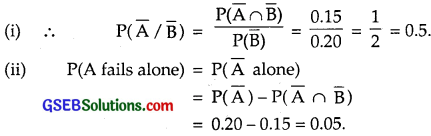Question 16.
Bag I contains 3 red and 4 black balls and Bag II contains 4 red and 5 black balls. One ball is transferred from Bag I to Bag II, then a ball is drawn from Bag II, the ball so drawn is found to be red in colour.
Find the probability that the transferred ball is black.
Solution:
Bag I contains 3 red and 4 black balls.
Bag II contains 4 red and 5 black balls.
Let E1 = Event that a red ball is drawn from Bag I
and E2 = Event that a black ball is drawn from Bag I.
∴ P(E1) = $$\frac{3}{7}$$, P(E2) = $$\frac{4}{7}$$.
After transferring a red ball from Bag I to Bag II, the Bag II will have 5 red and 5 black balls.
Let A be the event of drawing red ball.
∴ P(A/E1) = $$\frac{5}{10}$$ = $$\frac{1}{2}$$.
Further, when a black ball is transferred from Bag I to Bag II, it will contain 4 red and 6 black balls.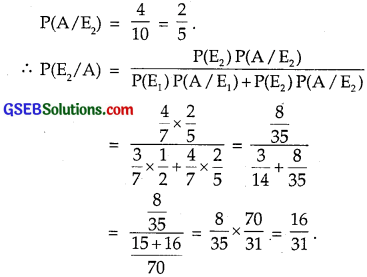Choose the correct answer in each of the following:

Question 17.
If A and B are two events, such that P(A) ≠ 0 and P(B/A) = 1, then
(A) A ⊂ B
(B) B ⊂ C
(C) B = ∅
(D) A = ∅
Solution:
P(B/A) = 0
⇒ $$\frac{P(A∩B)}{P(A)}$$ = 1
∴ P(A ∩ B) = P(A)
⇒ A ∩ B = A
⇒ A ⊂ B.
∴ Part (A) is the correct answer.Question 18.
If P(A/B) > P(A), then which of the following is correct:
(A) P(B/A) < P(B)
(B) P(A ∩ B) < P(A).P(B) (C) P(B/A) > P(B)
(D) P(B/A) = P(B)
Solution:
P(A/B) > P(A)
⇒ $$\frac{P(A∩B)}{P(B)}$$ > P(A)
∴ P(A ∩ B) > P(A).P(B)
or $$\frac{P(A∩B)}{P(A)}$$ > P(B)
⇒ P(B/A) > P(B).
∴ Part (C) is the correct answer.Question 19.
If A and B are any two events such that
P(A) + P(B) – P(A and B) = P(A), then:
(A) P(B/A) = 1
(B) P(A/B) = 1
(C) P(B/A) = 0
(D) P(A/B) = 0
Solution:
P(A) + P(B) – P(A ∩ B) = P(A)
P(B) – P(A ∩ B) = 0
or P(A ∩ B) = P(B)
or $$\frac{P(A∩B)}{P(B)}$$ = 1
i.e; P(A/B) = 1
∴ Part (B) is the correct answer.